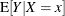Quantile Regression: The QUANTREG Procedure

The QUANTREG procedure models the effects of covariates on the conditional quantiles of a response variable by means of quantile regression.

Ordinary least squares regression models the relationship between one or more covariates X and the conditional mean of the response variable. Quantile regression extends the regression model to conditional quantiles of the response variable, such as the 90th percentile. Quantile regression is particularly useful when the rate of change in the conditional quantile, expressed by the regression coefficients, depends on the quantile. An advantage of quantile regression over least squares regression is its flexibility for modeling data with heterogeneous conditional distributions. Data of this type occur in many fields, including biomedicine, econometrics, and ecology.

Features that you will find in the QUANTREG procedure include the following:

• simplex, interior point, and smoothing algorithms for estimation

• sparsity, rank, and resampling methods for confidence intervals

• asymptotic and bootstrap methods to estimate covariance and correlation matrices of the parameter estimates

• Wald and likelihood ratio tests for the regression parameter estimates

• regression quantile spline fits